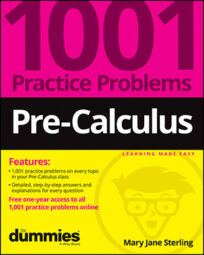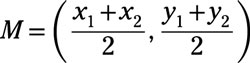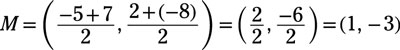##### Pre-Calculus: 1001 Practice Problems For Dummies (+ Free Online Practice)The basics of pre-calculus consist of reviewing number systems, properties of the number systems, order of operations, notation, and some essential formulas used in coordinate graphs. Vocabulary is important in mathematics because you have to relate a number or process to its exact description.

For pre-calculus, you’ll work with simplifying algebraic expressions and writing answers in the following ways:

• Identifying which are whole numbers, integers, and rational and irrational numbers

• Applying the commutative, associative, distributive, inverse, and identity properties

• Computing correctly using the order of operations (parentheses, exponents/powers and roots, multiplication and division, and then addition and subtraction)

• Graphing inequalities for the full solution

• Using formulas for slope, distance, and midpoint

• Applying coordinate system formulas to characterize geometric figures

Don’t let common mistakes trip you up; keep in mind that when working with simplifying expressions and communicating answers, your challenges will be

• Distributing the factor over every term in the parentheses

• Changing the signs of all the terms when distributing a negative factor

• Working from left to right when applying operations at the same level

• Assigning points to the number line in the correct order

• Placing the change in y over the change in x when using the slope formula

• Satisfying the correct geometric properties when characterizing figures

## Practice problems

1. Identify which property of numbers the equation illustrates. If x = 3 and y = x, then y = 3.

The transitive property says that if one value is equal to both a second value and a third, then the second and third values are equal to one another.

2. Find the midpoint of the segment between the points (−5, 2) and (7, −8).

Use the midpoint formula,and the given points: